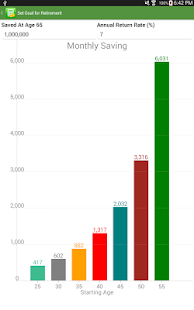Financial Calculators Pro

For phone and tablet, this application includes the complete package of financial calculators by Bishinews, a Google rated top developer:
Finance and Investment Calculators

For phone and tablet, this application includes the complete package of financial calculators by Bishinews, a Google rated top developer:

Finance and Investment Calculators
* TVM Calculator
* Currency Converter
* Compound Interest Calculator
* Return On Investment (ROI) Calculator
* IRR NPV Calculator
* MIRR Calculator
* Bond Calculator
* Tax Equivalent Yield Calculator
* Rule of 72 Calculator

Loan/Mortgage Calculators
* Loan Calculator
* Loan Comparison Calculator
* Loan Refinance Calculator
* APR Calculator
* Commercial Loan Calculator
* Loan Analysis Calculator
* Home Affordablility Calculator
* Mortgage Tax Saving Calculator
* Discount Points Calculator
* Fixed vs Adjustable Rate Calculator
* Bi-weekly Payment Calculator
* Interest Only Calculator

Retirement Calculators
* Retirement Planner
* 401k Contribution Calculator
* Retirement Savings Analysis
* Retirement Income Analysis
* Traditional IRA vs Roth IRA
* Required Minimum Distribution
* Social Security Estimator
* Asset Allocation Calculator
* Retirement Calculator

Stock Calculators
* Stock Return Calculator
* Stock Constant Growth Calculator
* Stock Non-constant Growth Calculator
* CAPM Calculator
* Expected Return Calculator
* Holding Period Return Calculator
* Weighted Average Cost of Capital Calculator
* Black-Scholes Option Calculator

Credit Card Calculators
* Credit Card Payoff Calculator
* Credit Card Minimum Calculator

Auto Loan and Lease Calculators
* Auto Loan Calculator
* Auto Lease Calculator

Miscellaneous Calculators
* Regular Calculator
* Tip Calculator
* Discount and Tax Calculator
* Percentage Calculator
* Date Calculator
* Unit Conversion
* US Inflation Calculator
* Margin and Markup Calculator
* Fuel Calculator
* Salary Increase Calculator
* US Paycheck Tax Calculator
* Net Distribution Calculator
* Financial Ratios
User can send the calculation results to others via Email. Financial Professionals can email the quote to their clients. The app allows you to edit and prioritize the list of calculators for easy access.

Screenshots

•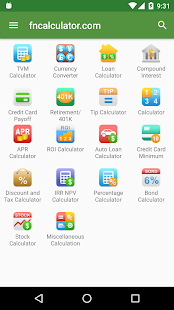•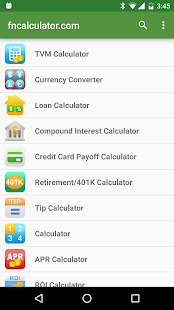•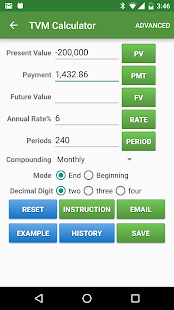•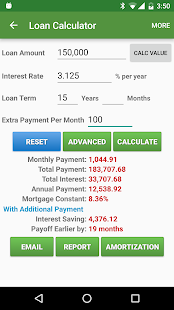•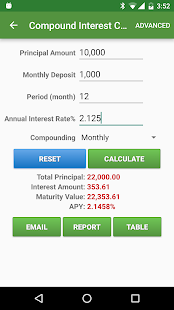•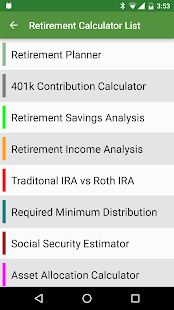•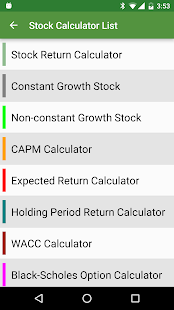•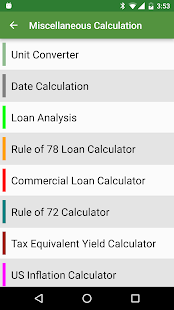•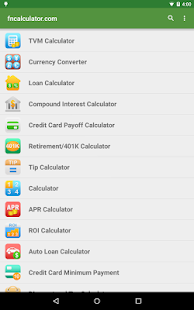•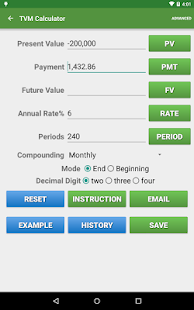•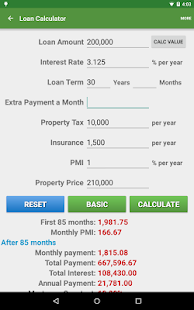•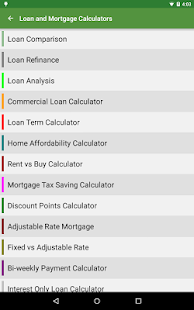•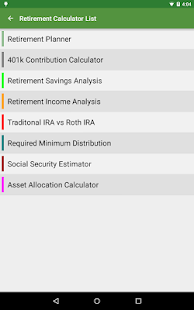•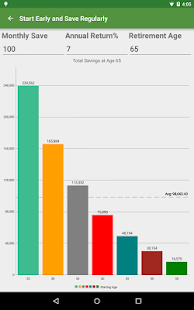•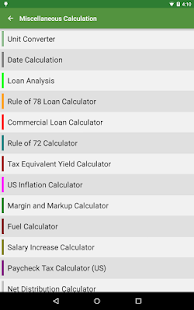•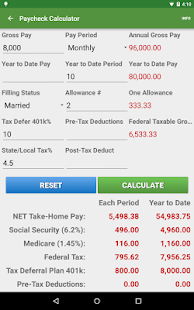•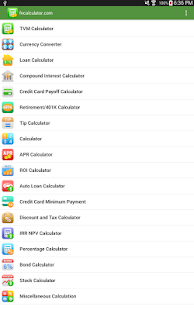•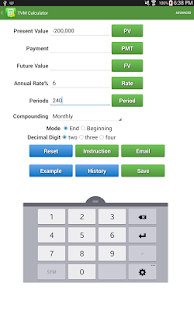•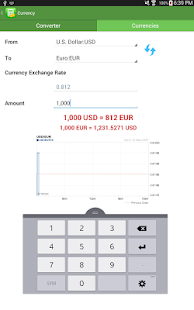•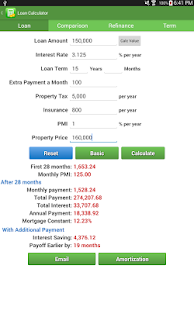•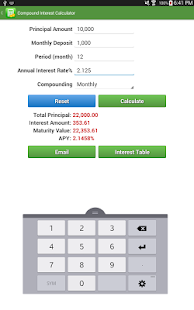•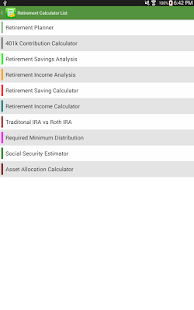•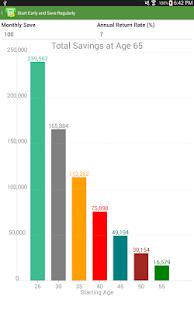•